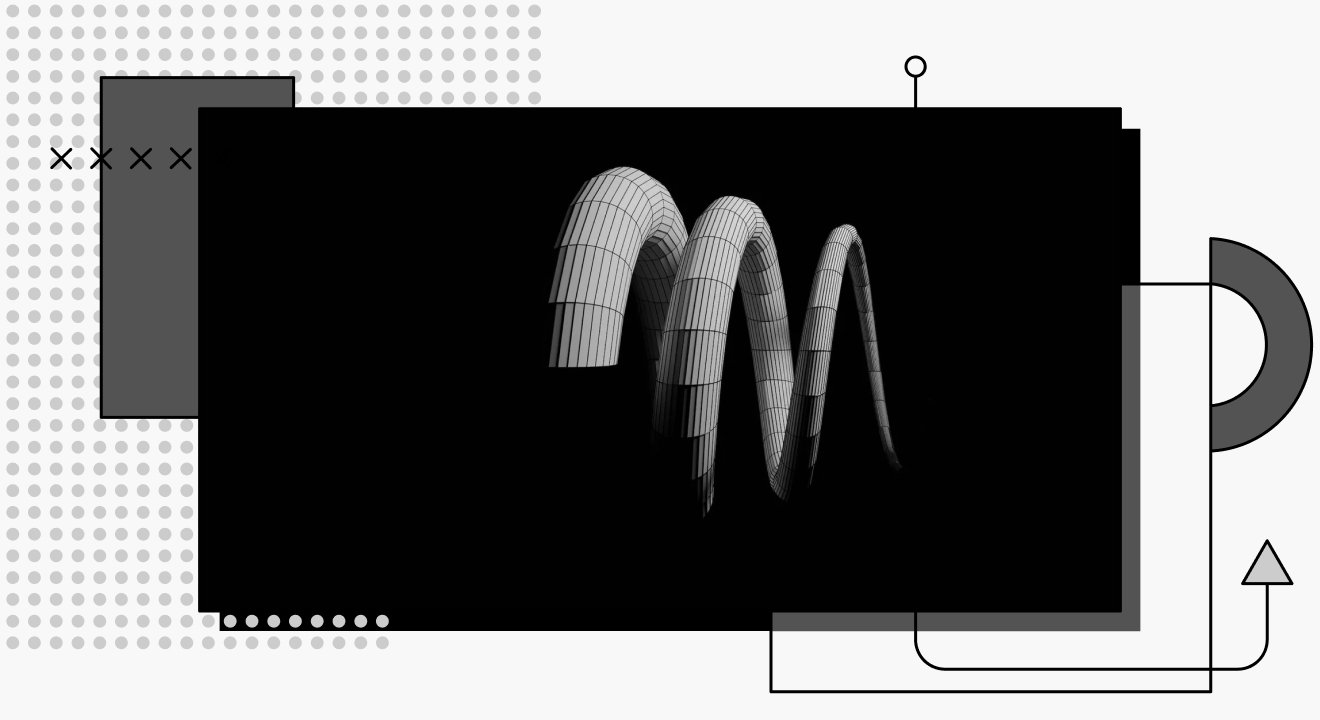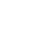# 3D Movement in Unity Using C#When developing a game, movement is usually one of the primary features implemented at the start of the project. For developers using Unity, there are multiple ways to achieve this functionality through code written using the C# programming language. This article will briefly summarize the various methods by showing code examples.

To be able to implement movement effectively with any of the listed ways, knowing how to use two specific Unity classes is of the utmost importance: the transform and rigidbody classes.

## Introduction to Transform

The transform class contains a game object's position, rotation and scale. Unity can use it to manipulate and store a game object's position, rotation, and scale. By default, all game objects in Unity come with a transform component.

There are some properties and methods that the transform class has, a few of which are discussed below.

### Properties

• `Transform.rotation`: Stores a quaternion, which Unity can use to manipulate the rotation of a game object or produce the current rotation.
• `Transform.position`: Used to get the position of a game object’s transform component and manipulate it to move the object in 3D world space.
• `Transform.localScale`: Allows developers to alter the size of a game object in the 3D world space.
• `Transform.localPosition`: Returns the position of the game object's transform component relative to its parent object.
• `Transform.forward`: Returns a normalized vector representing the z-axis in the 3D world space. Its value can be altered to cause a game object to move in the z-axis while also considering the rotation of the game object.

### Public Methods

• `Transform.SetParent()`:Sets the parent of the transform, but unlike the parent property, it allows the transform to keep its local orientation.
• `Transform.Translate()`: Moves the game object's transform in the direction and distance specified.
• `Transform.TransformDirection()`:Converts the direction (x,y,z) from local space to world space.
• `Transform.Rotate()`: Used to rotate a game object in multiple ways. The rotation produced is an Euler angle rather than quaternion.
• `Transform.RotateAround()`:Rotates a game object’s transform component about a fixed point.

For more information on the transform class with all its properties and methods, see the Unity Scripting API reference on Transforms.

## Introduction to Rigidbody

A rigidbody component added to a game object puts it under the influence of Unity's physics engine, which simulates real-life physics in the game project. Once the component is added to a game object in Unity, it is pulled downwards by gravity. It will react to any collision with other objects as long as the appropriate collider component is present. The rigidbody class can allow developers to apply forces to a game object in a way that seems physically real. Also, the rigidbody class has a lot of properties and methods, including the following:

### Properties

• `Rigidbody.drag`: Sets the drag of a game object.
• `Rigidbody.isKinematic`: Determines if a rigidbody attached to a game object is affected by physics.
• `Rigidbody.mass`: Specifies the mass of the rigidbody component.
• `Rigidbody.rotation`: Allows programmers to get and set the rotation of the rigidbody using Unity's physics engine.
• `Rigidbody.velocity`: Represents the velocity vector of the rigidbody. It is used to alter the rate of change of rigidbody position in world space.

### Methods

• `Rigidbody.AddExplosiveForce()`: Used to apply a force that simulates an explosion to a rigidbody.
• `Rigidbody.AddForce()`: Applies a force continuously to the rigidbody along the specified axis.
• `Rigidbody.AddTorque()`: Applies torque to the rigidbody component.
• `Rigidbody.Sleep()`: Forces a rigidbody to sleep for at least one frame.
• `Rigidbody.MovePosition()`: Causes the rigidbody to move to a specified position in the 3D world space.

For more information regarding rigidbodies and all of their properties and methods, see the Unity Scripting API Reference on Rigidbody.

## RigidBody Vs. Transforms

Although rigidbody seems similar to transform since some of their methods and properties perform the same functions, it would be wrong to presume they are the same. Rigidbody uses the built-in unity physics engine to simulate real-life physics, taking into account any collider attached to the game object. A transform doesn't use the physics engine; it is the in-game representation of a game object's location, size, and rotation.

## Five Ways to Code Movement

Unity can use one of the five methods or properties from the transform or rigidbody classes to implement the movement of a game object in Unity.

### Transform.Translate()

As we learned above, this method moves a game object's transform in the direction and distance specified. This section will discuss how to use it in a code block.

#### Declaration

`public void Translate(Vector3 translation);`

The parameter translation of datatype Vector3 specifies the direction in which the game object needs to move. By multiplying this parameter with a Time.deltaTime, we'll be able to achieve constant change in distance in the specified direction over time. Note that the frame rate of your PC determines this time.

To program a simple movement script using the translate function, let’s start by declaring four variables: a float for speed, two floats to store the vertical and horizontal movements of controls, and a vector3 for direction. The speed variable adjusts how fast the game object moves in the game world:

`private float speed = 10.0f;private float horizontalInput;private float verticalInput;private Vector3 movedirection;`

Next, we’ll initialize the directions by assigning inputs to each variable in the Update method:

`horizontalInput = Input.GetAxis(“Horizontal”);verticalInput = Input.GetAxis(“Vertical”);`

Now, let’s assign the horizontal and vertical variables to the `movedirection` variable:

`movedirection = new Vector3(horizontalInput , 0 ,verticalInput);`

We call the translate method and assign parameters:

`transform.Translate(movedirection * speed * Time.deltaTime);`

The entire code block is as so:

`using UnityEngine;using System.Collections;public class TestClass : MonoBehaviour{private float speed = 10.0f;private float horizontalInput;private float verticalInput;private Vector3 movedirection;   Void Update()   {        horizontalInput = Input.GetAxis(“Horizontal”);        VerticalInput = Input.GetAxis(“Vertical”);        movedirection = new Vector3(horizontalInput , 0 ,verticalInput);        transform.Translate(movedirection * speed * Time.deltaTime);|   }}`

Save to Pieces

### Transform.position

The position property is used to specify the location of a game object in the 3D world space, and it is accessible in the unity editor and through scripts. By steadily altering this value, we can achieve the movement mechanic.

#### Declaration

`public Vector3 position;`

To implement this in code, we’ll follow the first three steps in the `Transform.Translate() `section, and then add the following code in the `Update() `method:

`transform.position += movedirection * speed * Time.deltaTime;`

Here is the entire code block:

`using UnityEngine;using System.Collections;public class TestClass : MonoBehaviour{private float speed = 10.0f;private float horizontalInput;private float verticalInput;private Vector3 movedirection;    Void Update()    {         horizontalInput = Input.GetAxis(“Horizontal”);         VerticalInput = Input.GetAxis(“Vertical”);         movedirection = new Vector3(horizontalInput , 0 ,verticalInput);         transform.position += movedirection * speed * Time.deltaTime;    }}`

Save to Pieces

A previous section showed us that this method applies force to a game object using the built-in Unity physics engine. Next, we'll achieve controlled movement by integrating the inputs with this method and adjusting the amount of force applied using a variable.

#### Declaration

`public void AddForce(Vector3 force, Force mode mode);`

The force parameter is used to specify the direction and amount of force added in that direction, while the force mode dictates what type of force to apply. To use this method, we need to start by declaring and initializing several variables as well as a variable to store the `rigidbody`. It is important to note that before any code is written regarding the `rigidbody`, a `rigidbody` component must first be added to the game object to be used in the unity editor:

`private float force = 5.0f;private float horizontalInput;private float verticalInput;private Vector3 movedirection;private Rigidbody objRb;`

The `rigidbody` is initialized within the `Start()` method in the class:

`Start(){     objRb = GetComponent<Rigidbody>();}`

The `GetComponent<Rigidbody>()` code above fetches the Rigidbody from the game object that it is attached to. After initialization, we’ll call the `AddForce` method in the `FixedUpdate()` method:

`    void FixedUpdate()    {         objRb.AddForce(movedirection * force);    }`

The entire code block looks like this:

`using UnityEngine;using System.Collections;public class TestClass : MonoBehaviour{     private float force = 5.0f;     private float horizontalInput;     private float verticalInput;     private Vector3 movedirection;     private Rigidbody objRb;     void Start()     {          objRb = GetComponent<Rigidbody>();     }      void Update()     {          horizontalInput = Input.GetAxis(“Horizontal”);          verticalInput = Input.GetAxis(“Vertical”);          movedirection = new Vector3(horizontalInput , 0 ,verticalInput);     }     void FixedUpdate()     {          objRb.AddForce(movedirection * force);     }}`

Save to Pieces

### Rigidbody.velocity

This property represents the rate of change in a rigidbody's position. It gives a much quicker movement than the other listed methods in the rigidbody class.

Just like every other property or method in the rigidbody class, we must add a rigidbody component to the game object used in the project to use this property.

Let’s begin by declaring the following variables in the script file we’ve created:

`  private float horizontalInput;  private float verticalInput;  private Vector3 movedirection;  private Rigidbody objRb;  public float speed ;`

Next, we’ll initialize the inputs, rigidbody and movedirection.

`  void Start()  {       objRb = GetComponent<Rigidbody>();  }  void Update()  {       horizontalInput = Input.GetAxis(“Horizontal”);       verticalInput = Input.GetAxis(“Vertical”);       movedirection = new Vector3(horizontalInput , 0 ,verticalInput);  }`

Finally, let’s add the following code to the FixedUpdate() method:

`objRb.velocity = movedirection * speed;`

In the Unity editor, we can adjust the speed value once the script finishes compiling.

The entire code block looks like this:

`using UnityEngine;using System.Collections;public class TestClass : MonoBehaviour{     private float horizontalInput;     private float verticalInput;     private Vector3 movedirection;     private Rigidbody objRb;     public float speed ;     void Start()     {          objRb = GetComponent<Rigidbody>();     }     void Update()     {          horizontalInput = Input.GetAxis(“Horizontal”);          verticalInput = Input.GetAxis(“Vertical”);          movedirection = new Vector3(horizontalInput , 0 ,verticalInput);     }     void FixedUpdate()     {          objRb.velocity = movedirection * speed;     }}`

Save to Pieces

### Rigidbody.MovePosition()

Another way to program movement in Unity is by using this method call. It moves the kinematic rigidbody towards a specified position, factoring interpolation, thus creating a smooth transition between frames.

#### Declaration

`public void MovePosition(Vector3 position);`

The position parameter provides the new position for the rigidbody object. To illustrate this alternative means of coding movement, let’s look at a quick example below:

`using UnityEngine;using System.Collections;public class TestClass : MonoBehaviour{     Vector3 movedirection;     float horizontalInput;     float verticalInput;     Rigidbody m_Rigidbody;     public float speed = 5f ;     void Start()     {          objRb = GetComponent<Rigidbody>();     }     void FixedUpdate()     {          horizontalInput = Input.GetAxis(“Horizontal”);          verticalInput = Input.GetAxis(“Vertical”);          movedirection = new Vector3(horizontalInput , 0 ,verticalInput);          m_Rigidbody.MovePosition(transform.position + movedirection * Time.deltaTime * speed);     }}`

Save to Pieces

## Conclusion

This article divulged several ways to code a movement mechanic in Unity with C#. Keep in mind, however, that one is not necessarily superior to the other. It all depends on the kind of movement you'd like to achieve and whether or not you'd like to use Unity's built-in physics engine for your interactions, or have a custom physics system.